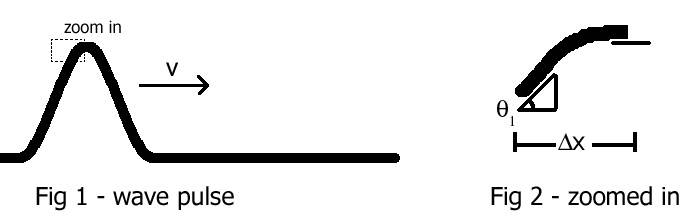# Wave Pulse - Finding Average Transverse Acceleration of Segment

ArtVandelay

## Homework Statement

A travelling wave pulse is shown in figure 1 below, travelling at v=6 m/s across a string. In figure 2, a short segment of the string is shown zoomed in. The angle of this string goes from θ1 = 17o to zero within a small horizontal distance Δx = 3 mm.## Homework Equations

∂^2y/∂x^2= 1/v2 * (∂^2y/∂t2^2)

## The Attempt at a Solution

I know using that equation I want to solve for the acceleration, which is the second derivative of position (y), so I should solve for (∂^2y/∂t2^2) in terms of v, but when I do that I am still left with an equation in terms of ∂^2y/∂x^2, the concavity, which I am not sure what do do with / what it equals.

So next I tried using my intuition:

|average transverse acceleration| = |Δv|/Δt = initial transverse velocity / Δt.

And since initial transverse velocity = the slope of the graph at point 1 (where Δx begins) = rise/run = tan(θ), and Δt = Δx/v, the above eqn becomes:

( v * tan(θ) ) / Δx

But that isn't right